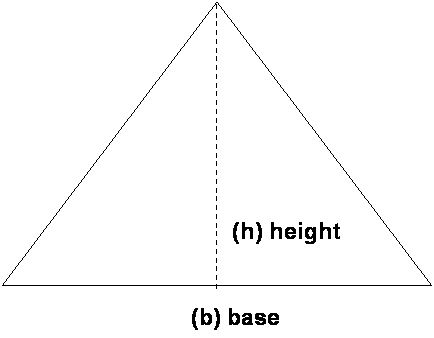Area of a Square              Area of a Rectangle              Area of a TriangleArea of a Square              Area of a Rectangle              Area of a Triangle   AREA of a TRIANGLE = (.5) times the BASE  times the HEIGHT. A = (.5)(b)h)   Find the area for the following triangles:   1) A triangle with a base of 4 yds. and a height of 3 yds.   A=6yds2           2) A triangle with a base of 8 mm and a height of 16 mm.     A=64mm2         3) A triangle with a base of 4x and a height of 3x.     A=6x2